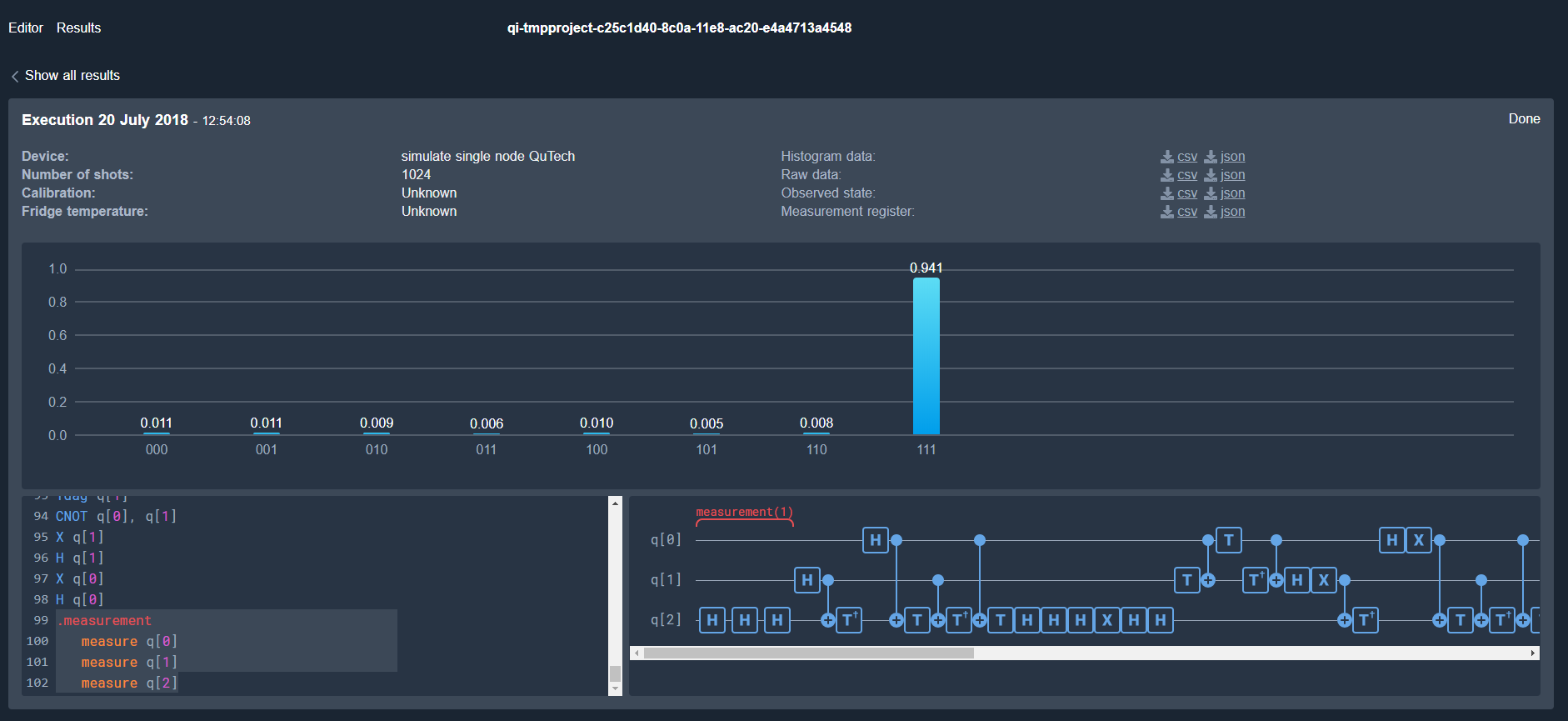# Grover Search Algorithm¶

This notebook is an adapted version from https://github.com/QISKit/qiskit-tutorial. We show to perform the Grover Search algorithm both on a local simulator and on the Quantum Inspire backend.

For more information about how to use the IBM Q Experience (QX), consult the tutorials, or check out the community.

Contributors Pieter Eendebak, Giacomo Nannicini and Rudy Raymond (based on this article)

## Introduction¶

Grover search is one of the most popular algorithms used for searching a solution among many possible candidates using Quantum Computers. If there are $N$ possible solutions among which there is exactly one solution (that can be verified by some function evaluation), then Grover search can be used to find the solution with $O(\sqrt{N})$ function evaluations. This is in contrast to classical computers that require $\Omega(N)$ function evaluations: the Grover search is a quantum algorithm that provably can be used search the correct solutions quadratically faster than its classical counterparts.

Here, we are going to illustrate the use of Grover search to find a particular value in a binary number. The key elements of Grovers algorithm are:

1. Initialization to a uniform superposition
2. The oracle function
3. Reflections (amplitude amplification)

## The oracle function¶

We implement an oracle function (black box) that acts as -1 on a single basis state, and +1 on all other status.

Another important procedure in Grover search is to have an operation that perfom the inversion-about-the-average step, namely, it performs the following transformation:

$$\sum_{j=0}^{2^{n}-1} \alpha_j |j\rangle \rightarrow \sum_{j=0}^{2^{n}-1}\left(2 \left( \sum_{k=0}^{k=2^{n}-1} \frac{\alpha_k}{2^n} \right) - \alpha_j \right) |j\rangle$$

The above transformation can be used to amplify the probability amplitude $\alpha_s$ when s is the solution and $\alpha_s$ is negative (and small), while $\alpha_j$ for $j \neq s$ is positive. Roughly speaking, the value of $\alpha_s$ increases by twice the average of the amplitudes, while others are reduced. The inversion-about-the-average can be realized with the sequence of unitary matrices as below:

$$H^{\otimes n} \left(2|0\rangle \langle 0 | - I \right) H^{\otimes n}$$

The first and last $H$ are just Hadamard gates applied to each qubit. The operation in the middle requires us to design a sub-circuit that flips the probability amplitude of the component of the quantum state corresponding to the all-zero binary string. The sub-circuit can be realized by the following function, which is a multi-qubit controlled-Z which flips the probability amplitude of the component of the quantum state corresponding to the all-one binary string. Applying X gates to all qubits before and after the function realizes the sub-circuit.

Finally, the inversion-about-the-average circuit can be realized by the following function:

We show the circuit that performs inversion about the average on $n$ qubits. We also show the effect of the circuit on the basis states.

## Grover Search: putting all together¶

The complete steps of Grover search is as follow.

1. Create the superposition of all possible solutions as the initial state (with working qubits initialized to zero) $$\sum_{j=0}^{2^{n}-1} \frac{1}{2^n} |j\rangle |0\rangle$$
2. Repeat for $T$ times:

• Apply the blackbox function
3. Measure to obtain the solution

Before we go to the code to perform the Grover search we make some remarks on the number of repetitions $T$ that we have to perform (for details see Grover algorithm, Wikipedia).

Each Grover step rotates the 'winner solution' by a fixed angle. This means that after a certain number of steps we arrive at the optimal approximation (e.g. the amplitude of the winner solution is maximal). If we then apply more iterations, the quality of our result will go down. For a database of size $N=2^n$ the optimal number of iterations is $$r=\pi \sqrt{N}/4$$

The probablity of the winner state after $T$ iterations is $\sin( (T+1/2)\theta)^2$

Finally we define the complete circuit for Grovers algorithm, excute it and show the results.

As expected, the state that is indicated by the oracle function has the highest probability of begin measured.

We show the full circuit that was generated by the code.

## Run the circuit on the Quantum Inspire simulator¶

First we make a connection to the Quantum Inspire website.

We can list backends and perform other functions with the QuantumInspireProvider.

We create a QisKit backend for the Quantum Inspire interface and execute the circuit generated above.

We can wait for the results and then print them

Visualization can be done with the normal Python plotting routines, or with the QisKit SDK.

A screenshot from the execution result on the Quantum Inspire website."A fast quantum mechanical algorithm for database search", L. K. Grover, Proceedings of the 28th Annual ACM Symposium on the Theory of Computing (STOC 1996)

 "Tight bounds on quantum searching", Boyer et al., Fortsch.Phys.46:493-506,1998

 "Quantum Inspire"